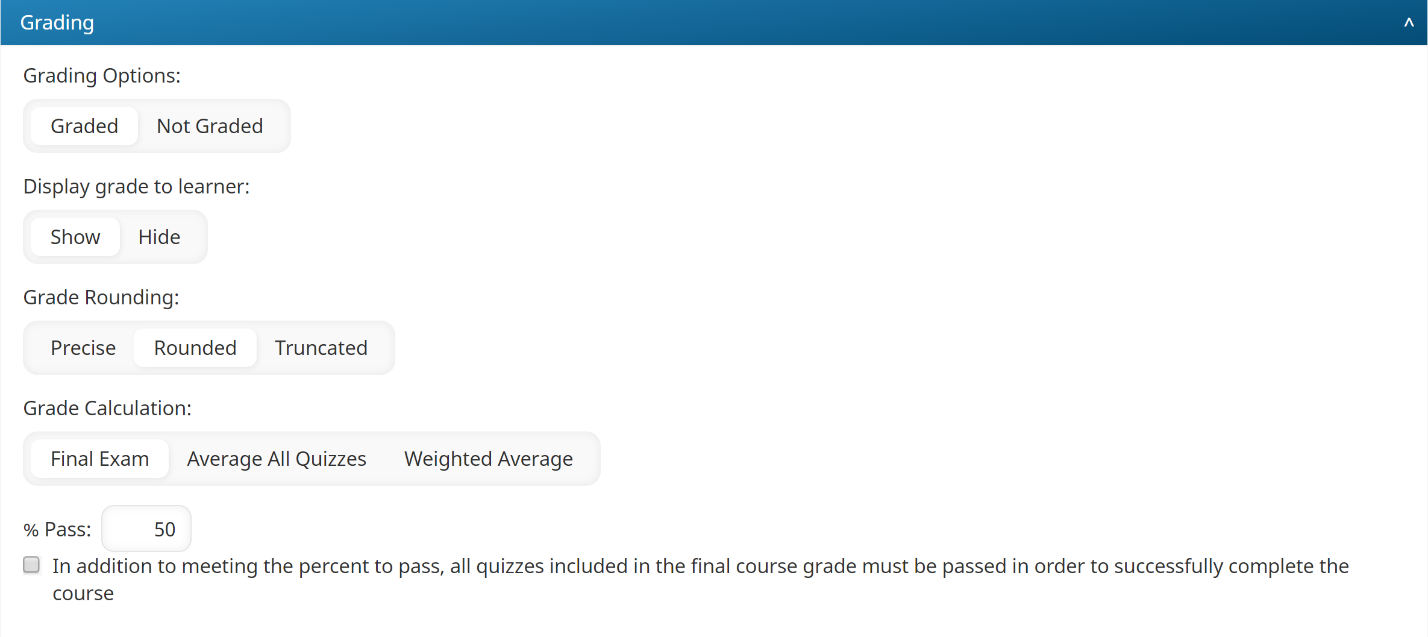• Dark
Light

• Dark
Light

Article Summary

These online course settings are located in the Grading accordion of the Course Information workscreen.## Settings

Be sure to click Save after changing these settings.

Select one of the following options:

• Graded - A final mark is recorded based on the selected Grade Calculation setting.
• Not Graded - No final mark is recorded for learners.

This setting is only available when the Grading Options setting is set to Graded. The Display Grade to Learner setting controls whether the course certificate, course/quiz grade(s), pass/fail indicator, and transcript are visible on the learner interface.

• Show - The course certificate, grade(s), pass/fail indicator, and transcript are visible to the learner.
• Hide - The course certificate, grade(s), pass/fail indicator, and transcript are not visible to the learner.

Determines how the course grade is rounded.

• Precise - Rounds the grade to 2 decimal places. For example, a grade of 87.754% would be rounded to 87.75%.
• Rounded - Rounds the grade to the nearest whole number. For example, a grade of 87.7% would be rounded to 88%.
• Truncated - Removes decimals from the grade. For example, a grade of 87.7% would be rounded to 87%.

This setting is only available when the Grading Options setting is set to Graded. It determines how the learner's course grade is calculated.

• Final Exam - The mark from the learner's last quiz is recorded as their course grade. If you select this option, the last task in the course must be a quiz.
• Average All Quizzes - Averages the learner's quiz marks to calculate their course grade. Only quizzes that have the Include Grade In Final Course Grade Calculation setting checked are included in the calculation.

• 25%
• 50%
• 75%

The course grade would be 50% calculated by (25+50+75)/3.

When calculating a weighted average, only the last quiz attempt is used for each quiz.

• 5/20
• 5/10
• 30/40

The course grade would be 57% calculated by (5+5+30)/(20+10+40).

### Percent to Pass

This setting is only available when the Grade Calculation setting is set to Average All Quizzes or Weighted Average. The Percent to Pass setting is the minimum course grade percentage the learner must have to pass the course.

### All Graded Quizzes Must Pass

This setting is only available when the Grade Calculation setting is set to Average All Quizzes or Weighted Average. Selecting the All Graded Quizzes Must Pass setting requires the learner to pass all quizzes in order to pass the course.

For example, suppose a course grade is calculated using an average of all quizzes and that the minimum required percentage is 70%. Suppose a learner in the course had the following quiz grades:

• 85% (pass)
• 60% (fail)
• 75% (pass)

In this example, the average of all quizzes is 73% which is above the minimum required to pass the class. However, because the learner did not pass all quizzes, the learner will not pass the course.